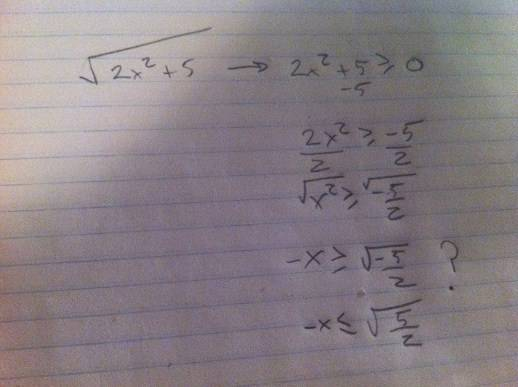# Finding the domain of a composite function help.

nukeman

## Homework Statement

Ok, I just worked out a composite function, and it left me with:

√2x^2+5)

Now, how do I find the domain from that? I don't understand that my text says the domain of that is just all Real numbers ???

What makes this different than other square functions that we are able to find the domain like: √2-x ?

## Answers and Replies

Villyer
This isn't any different from those. How would you go about finding the domain of the example function you posted?

nukeman
Then why did my book say the domain is all REAL numbers?

I would go:Mentor
You did a bunch of work that doesn't get you anywhere.

The square of any real number is ≥ 0.

IOW, x2 ≥ 0, for all real x
so 2x2 ≥ 0, for all real x
so 2x2 + 5 ≥ 5, for all real x

nukeman
Kinda lost here.

So, 2x^2 + 5

the 2x^2 part is always the same, as 2x^2, x can be any real number. And why is that?

how did you get 2x^2 + 5 <= 5?

What would the domain be?

Thanks Mark!!

Saitama
x2 is always positive, do you expect 2x2 or 2x2+5 to result a negative number?
Kinda lost here.

So, 2x^2 + 5

the 2x^2 part is always the same, as 2x^2, x can be any real number. And why is that?
x can be any real number because square of every real number is defined.

nukeman
Oh....ok

So, everytime I get a 2x^2 in a square root, and have to find the domain, its just all real numbers? Then I just worry about what after it, like the +5?

But then, wouldnt 2x^2 + 5 be x >= -5?

Mentor
Kinda lost here.

So, 2x^2 + 5
This is not a complete statement. So 2x2 + 5 is what?
the 2x^2 part is always the same, as 2x^2, x can be any real number. And why is that?

how did you get 2x^2 + 5 <= 5?
I didn't. I got 2x2 + 5 ≥ 5
What would the domain be?

Thanks Mark!!

Oh....ok

So, everytime I get a 2x^2 in a square root, and have to find the domain, its just all real numbers? Then I just worry about what after it, like the +5?
If you have an expression like x2, or 2x2 or .1x2, it is always guaranteed to be ≥ 0, because the square of any real number can't be negative.
But then, wouldnt 2x^2 + 5 be x >= -5?
NO!

2x2 + 5 is an expression that has a value for each value of x.

x ≥ -5,
2x2 + 5 = 0,
2x2 + 5 < 0,
and
2x2 + 5 ≥ 0
are all statements that are either true or false, possibly depending on the value of x, but possibly not.

You can't compare 2x2 + 5 (an expression) and x ≥ -5 (a statement).

2x2 + 5 ≥ 0,

you can add -5 to both sides to get
2x2 ≥ -5

This is legitimate, but unwise. Since x2 ≥ 0 for all real x, then 2x2 ≥ 0 for all x, so 2x2 is automatically ≥ -5.

nukeman
Oh ok, I see what you mean here. Thanks Mark.

So, for that expression, if they ask for the domain of that expression, what would it be? That is where I am lost.

Mentor
By that expression, I assume you mean ## \sqrt{2x^2 + 5}##, right? I don't see how you can still be asking this. You know the answer, and this thread has explained what's going on in considerable detail.